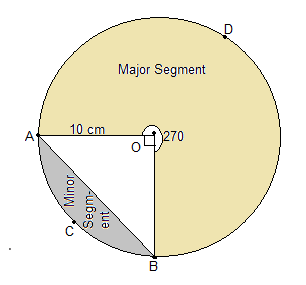Newbie

# A chord of a circle of radius 10 cm subtends a right angle at the centre. Find the area of the corresponding: (i) minor segment (ii) major sector. (Use π = 3.14) Q.4(1),(2)

• 0

How i solve the ncert class 10 chapter areas related to circle of exercise 12.2 question no. 4 . Find the best and easiest way to solve the question of this chapter . Give me the simplest solution of this question.A chord of a circle of radius 10 cm subtends a right angle at the centre. Find the area of the corresponding: (i) minor segment (ii) major sector. (Use π = 3.14)

Share

1.Here AB be the chord which is subtending an angle 90° at the center O.

It is given that the radius (r) of the circle = 10 cm

(i) Area of minor sector = (90/360°)×πr2

= (¼)×(22/7)×102

Or, Area of minor sector = 78.5 cm2

Also, area of ΔAOB = ½×OB×OA

Here, OB and OA are the radii of the circle i.e. = 10 cm

So, area of ΔAOB = ½×10×10

= 50 cm2

Now, area of minor segment = area of minor sector – area of ΔAOB

= 78.5 – 50

= 28.5 cm2

(ii) Area of major sector = Area of circle – Area of minor sector

= (3.14×102)-78.5

= 235.5 cm2

• 0